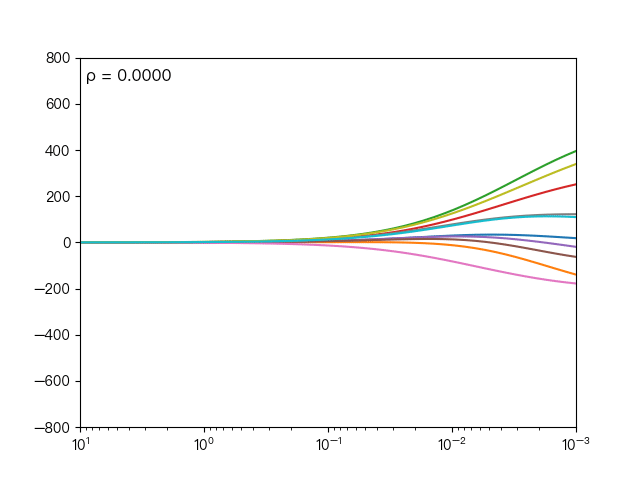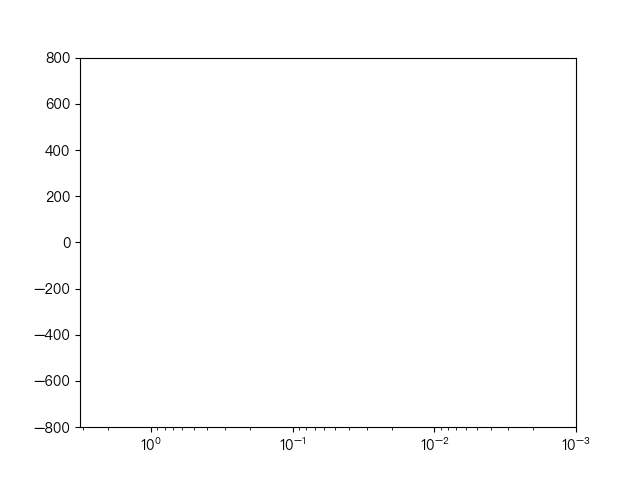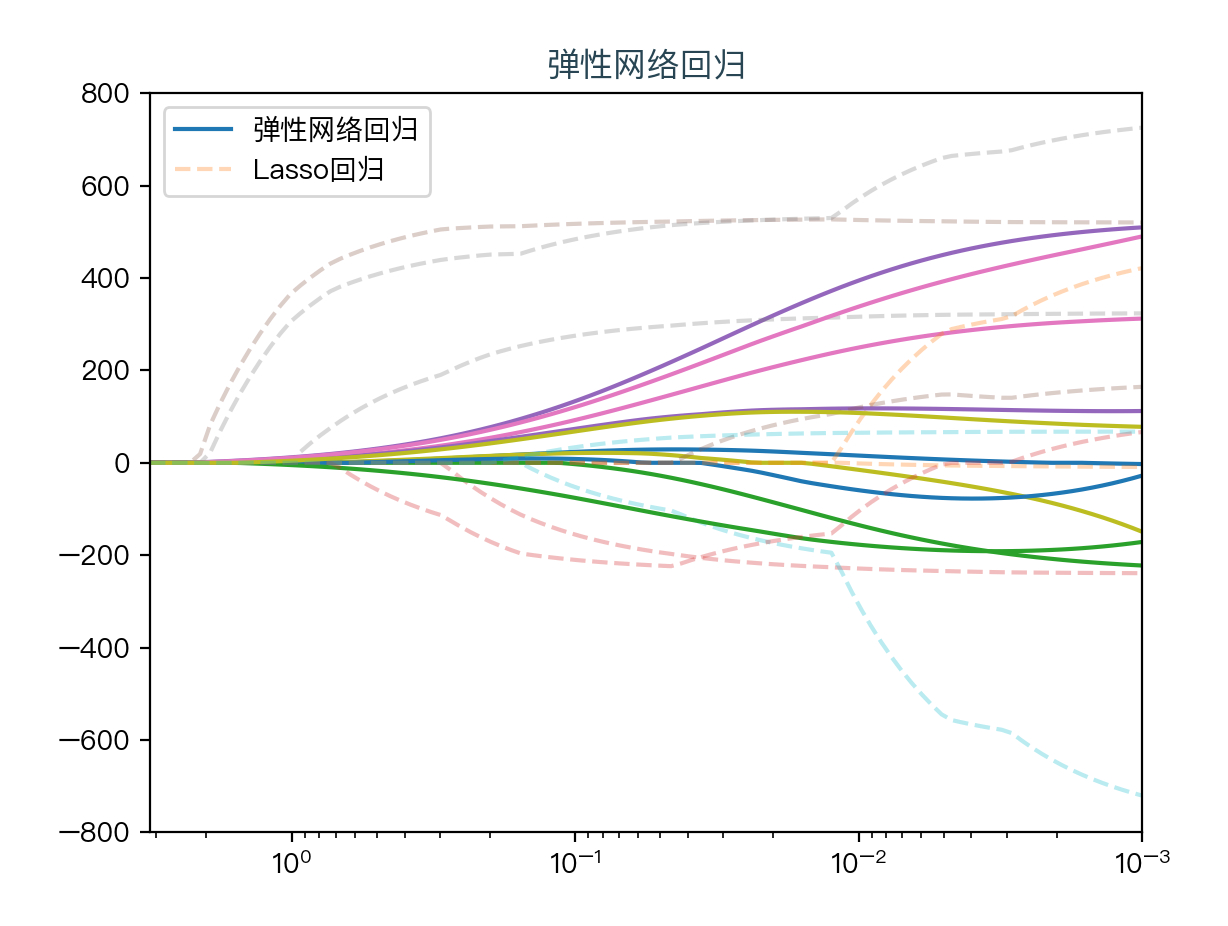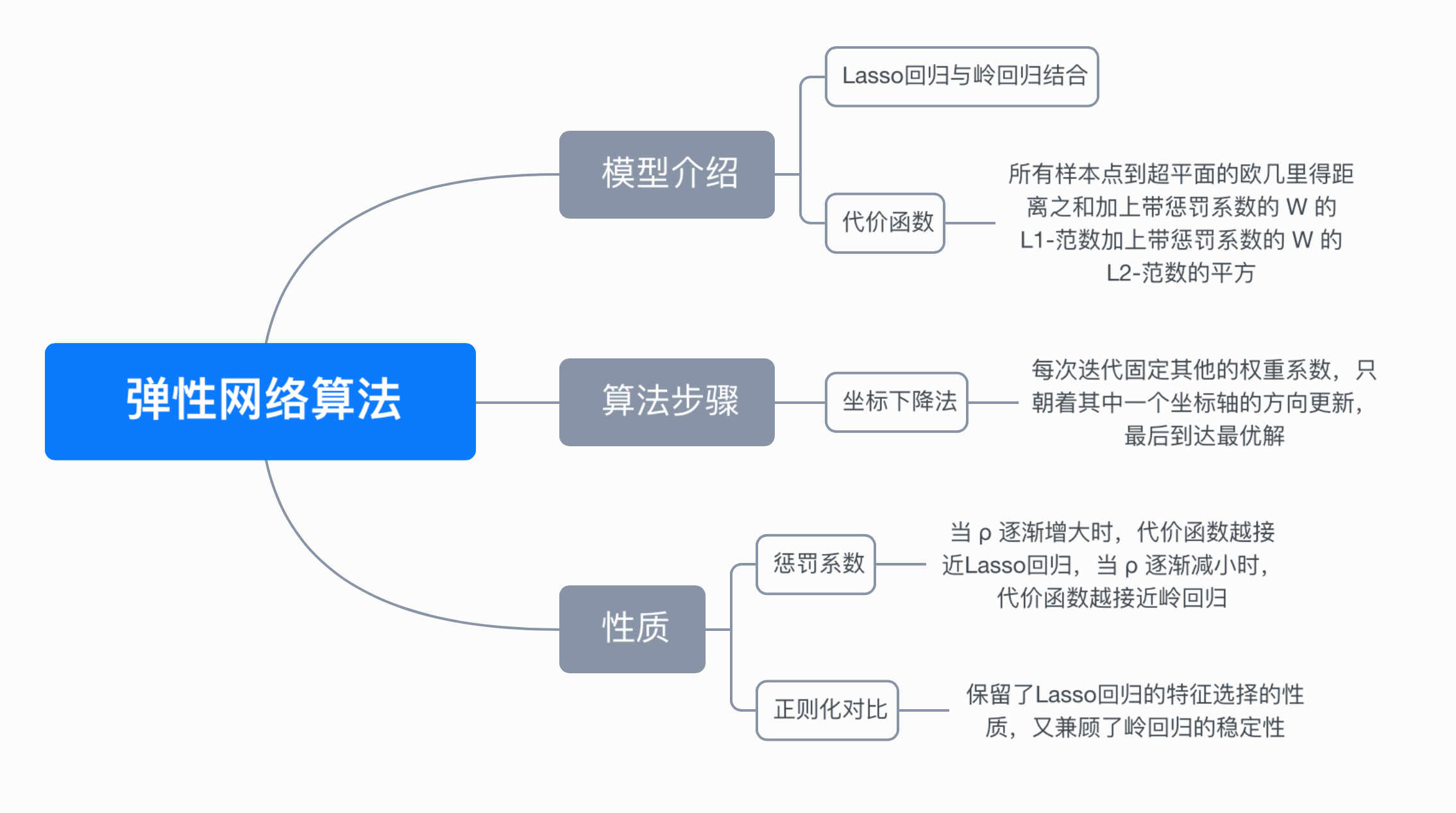• 主要介绍了用tensorflow实现弹性网络回归算法
• 弹性网络回归 弹性网络ElasticNet是同时使用了系数向量的 l1 范数和 l2 范数的线性回归模型，使得可以学习得到类似于Lasso的一个稀疏模型，同时还保留了 Ridge 的正则化属性，结合了二者的优点，尤其适用于有多个...

弹性网络回归

弹性网络ElasticNet是同时使用了系数向量的 l1 范数和 l2 范数的线性回归模型，使得可以学习得到类似于Lasso的一个稀疏模型，同时还保留了 Ridge 的正则化属性，结合了二者的优点，尤其适用于有多个特征彼此相关的场合。

主要参数说明

alpha: a值。

fit_intercept:一个布尔值，指定是否需要计算b值。如果为False，那么不计算b值（模型会认为你已经将数据中心化了）。

max_iter:整数值，指定最大迭代次数。

normalize：一个布尔值。如果为True，那么训练样本会在回归之前被归一化。

copy_X：一个布尔值，如果为True，则会复制X值

precompute：一个布尔值或者一个序列。他决定是否提前计算Gram矩阵来加速计算。

tol：一个浮点数，指定判断迭代收敛与否的阈值。

warm_start：一个布尔值，如为True，那么使用前一次训练结果继续训练。否则重头开始训练。

positive：一个布尔值，如为Ture，那么强制要求全中响亮的分量都为整数。

selection：一个字符串，可以为‘cyclic’（更新时候，从前向后一次选择权重向量的一个分量来更新）或者‘random’（随机选择权重向量的一个分量来更新），他指定了当每轮迭代的时候，选择权重向量的一个分量来更新

random_state：一个整数或者一个RandomState实例，或者为None。如果为整数，则他指定了随机数生成器种子。如果为RandomState实例，则指定了随机数生成器。如果为None，则使用默认的随机数生成器。

%config InteractiveShell.ast_node_interactivity = 'all'   #同时输出多行结果
from sklearn.linear_model import ElasticNet
reg = ElasticNet(alpha=1.0, l1_ratio=0.7)
X = [, ]
y = [1, 2]
reg.fit(X, y)
ElasticNet(alpha=1.0, copy_X=True, fit_intercept=True, l1_ratio=0.7,
max_iter=1000, normalize=False, positive=False, precompute=False,
random_state=None, selection='cyclic', tol=0.0001, warm_start=False)   #参数含义和lasso和ridge类似
reg.predict([])
reg.coef_
reg.intercept_

ElasticNet(alpha=1.0, copy_X=True, fit_intercept=True, l1_ratio=0.7,
max_iter=1000, normalize=False, positive=False, precompute=False,
random_state=None, selection='cyclic', tol=0.0001, warm_start=False)

ElasticNet(alpha=1.0, copy_X=True, fit_intercept=True, l1_ratio=0.7,
max_iter=1000, normalize=False, positive=False, precompute=False,
random_state=None, selection='cyclic', tol=0.0001, warm_start=False)

array([1.54198473])
array([0.08396947])
1.0381679389312977

#修改参数比较结果
reg = ElasticNet(alpha=1.0, l1_ratio=0.3)   # 修改参数，进行对比
reg.fit(X, y)
ElasticNet(alpha=1.0, copy_X=True, fit_intercept=True, l1_ratio=0.3,
max_iter=1000, normalize=False, positive=False, precompute=False,
random_state=None, selection='cyclic', tol=0.0001, warm_start=False)
reg.predict([])
reg.coef_
reg.intercept_

ElasticNet(alpha=1.0, copy_X=True, fit_intercept=True, l1_ratio=0.3,
max_iter=1000, normalize=False, positive=False, precompute=False,
random_state=None, selection='cyclic', tol=0.0001, warm_start=False)

ElasticNet(alpha=1.0, copy_X=True, fit_intercept=True, l1_ratio=0.3,
max_iter=1000, normalize=False, positive=False, precompute=False,
random_state=None, selection='cyclic', tol=0.0001, warm_start=False)

array([1.56834532])
array([0.13669065])
0.748201438848921

#利用IRIS数据集进行弹性网络回归
import pandas  as pd
import numpy  as np
from sklearn import linear_model
from sklearn import metrics
#导入IRIS数据集
#特征矩阵
X=iris.data
#目标向量
y=iris.target
from sklearn.cross_validation import train_test_split #导入数据划分包
#以20%的数据构建测试样本，剩余作为训练样本
X_train,X_test,y_train,y_test=train_test_split(X,y,test_size=0.20,random_state =1)
elastic= linear_model.ElasticNet(alpha=0.1,l1_ratio=0.5)  # 设置lambda值,l1_ratio值
elastic.fit(X_train,y_train)  #使用训练数据进行参数求解
y_hat2 = elastic.predict(X_test)  #对测试集的预测
print ("RMSE:", np.sqrt(metrics.mean_squared_error(y_test, y_hat2)))  #计算RMSE

ElasticNet(alpha=0.1, copy_X=True, fit_intercept=True, l1_ratio=0.5,
max_iter=1000, normalize=False, positive=False, precompute=False,
random_state=None, selection='cyclic', tol=0.0001, warm_start=False)

RMSE: 0.25040264500501913

展开全文弹性网络回归
• : [158.41] [ 34 0 170 165 170 165 170 165] : [176.03076923] 岭回归、lasso回归、弹性网络回归预测结果比较 #以iris数据为例 #导入基本程序包 import pandas as pd import numpy as np from sklearn.datasets ...

预测儿童身高案例

案例背景介绍

理论上，一个人的身高除了随年龄变大而增长之外，在一定程度上还受到遗传和饮食习惯以及其他因素的影响。在这里我们把问题简化一下，假定一个人的身高只受年龄、性别、父母身高、祖父母身高和外祖父母身高这几个因素的影响，并假定大致符合线性关系。

%config InteractiveShell.ast_node_interactivity = 'all'   #同时输出多行结果
import copy
import numpy as np
from sklearn import linear_model
# 训练数据，每一行表示一个样本，包含的信息分别为：
# 儿童年龄,性别（0女1男）
# 父亲、母亲、祖父、祖母、外祖父、外祖母的身高
x = np.array([[1, 0, 180, 165, 175, 165, 170, 165],
[3, 0, 180, 165, 175, 165, 173, 165],
[4, 0, 180, 165, 175, 165, 170, 165],
[6, 0, 180, 165, 175, 165, 170, 165],
[8, 1, 180, 165, 175, 167, 170, 165],
[10, 0, 180, 166, 175, 165, 170, 165],
[11, 0, 180, 165, 175, 165, 170, 165],
[12, 0, 180, 165, 175, 165, 170, 165],
[13, 1, 180, 165, 175, 165, 170, 165],
[14, 0, 180, 165, 175, 165, 170, 165],
[17, 0, 170, 165, 175, 165, 170, 165]])

# 儿童身高，单位：cm
y = np.array([60, 90, 100, 110, 130, 140, 150, 164, 160, 163, 168])

# 创建线性回归模型
lr = linear_model.LinearRegression()
# 根据已知数据拟合最佳直线
lr.fit(x, y)

LinearRegression(copy_X=True, fit_intercept=True, n_jobs=1, normalize=False)

# 待测的未知数据，其中每个分量的含义和训练数据相同
xs = np.array([[10, 0, 180, 165, 175, 165, 170, 165],
[17, 1, 173, 153, 175, 161, 170, 161],
[34, 0, 170, 165, 170, 165, 170, 165]])

for item in xs:
# 为不改变原始数据，进行深复制，并假设超过18岁以后就不再长高了
# 对于18岁以后的年龄，返回18岁时的身高
item1 = copy.deepcopy(item)
if item1 > 18:
item1 = 18
print(item, ':', lr.predict(item1.reshape(1,-1)))

[ 10   0 180 165 175 165 170 165] : [140.56153846]
[ 17   1 173 153 175 161 170 161] : [158.41]
[ 34   0 170 165 170 165 170 165] : [176.03076923]

岭回归、lasso回归、弹性网络回归预测结果比较

#以iris数据为例
#导入基本程序包
import pandas  as pd
import numpy  as np
from sklearn import linear_model
from sklearn import metrics
#导入IRIS数据集
#特征矩阵
X=iris.data
#目标向量
y=iris.target
from sklearn.cross_validation import train_test_split #导入数据划分包
#以20%的数据构建测试样本，剩余作为训练样本
X_train,X_test,y_train,y_test=train_test_split(X,y,test_size=0.20,random_state =1)
elastic= linear_model.ElasticNet(alpha=0.1,l1_ratio=0.5)  # 设置lambda值,l1_ratio值 ，这里需要注意的是，ElasticNet最基本的参数是alpha、l1_ratio，alpha其正则化选项中的λ，l1_ratio则是对应α。这里的random_state就是为了保证程序每次运行都分割一样的训练集和测试集。
elastic.fit(X_train,y_train)  #使用训练数据进行参数求解
y_hat2 = elastic.predict(X_test)  #对测试集的预测
print ("RMSE:", np.sqrt(metrics.mean_squared_error(y_test, y_hat2)))  #计算RMSE

ElasticNet(alpha=0.1, copy_X=True, fit_intercept=True, l1_ratio=0.5,
max_iter=1000, normalize=False, positive=False, precompute=False,
random_state=None, selection='cyclic', tol=0.0001, warm_start=False)
RMSE: 0.25040264500501913

比较三种回归的不同

岭回归

ridge= linear_model.Ridge(alpha=0.1)  # 设置lambda值
ridge.fit(X_train,y_train)  #使用训练数据进行参数求解
y_hat = ridge.predict(X_test)  #对测试集的预测
from sklearn import metrics  #导入metrics评价模块
print ("RMSE:", np.sqrt(metrics.mean_squared_error(y_test, y_hat)))  #计算RMSE

Ridge(alpha=0.1, copy_X=True, fit_intercept=True, max_iter=None,
normalize=False, random_state=None, solver='auto', tol=0.001)
RMSE: 0.24949141419802737

lasso回归

lasso= linear_model.Lasso(alpha=0.1)  # 设置lambda值
lasso.fit(X_train,y_train)  #使用训练数据进行参数求解
y_hat1 = lasso.predict(X_test)  #对测试集的预测
print ("RMSE:", np.sqrt(metrics.mean_squared_error(y_test, y_hat1)))  #计算RMSE

Lasso(alpha=0.1, copy_X=True, fit_intercept=True, max_iter=1000,
normalize=False, positive=False, precompute=False, random_state=None,
selection='cyclic', tol=0.0001, warm_start=False)

RMSE: 0.2555801316884705

#得到岭回归的RMSE: 0.249491414198027；得到Lasso回归的RMSE: 0.2555801316884705
#我们看到，在相同的lambda值下，ElasticNet回归的RMSE介于岭回归、lasso之间，即比Lasso回归的效果好，比岭回归的效果差。
#当然我们可以调整参数，会得到不同结果。

展开全文lasso 岭回归 弹性网络
• 机器学习算法系列（六）- 弹性网络回归算法（Elastic Net Regression Algorithm）

阅读本文需要的背景知识点：岭回归、Lasso回归、一点点编程知识

一、引言

前面学习了岭回归与Lasso回归两种正则化的方法，当多个特征存在相关时，Lasso回归可能只会随机选择其中一个，岭回归则会选择所有的特征。这时很容易的想到如果将这两种正则化的方法结合起来，就能够集合两种方法的优势，这种正则化后的算法就被称为弹性网络回归1 （Elastic Net Regression）

二、模型介绍

弹性网络回归算法的代价函数结合了Lasso回归和岭回归的正则化方法，通过两个参数 λ 和 ρ 来控制惩罚项的大小。

Cost ⁡ ( w ) = ∑ i = 1 N ( y i − w T x i ) 2 + λ ρ ∥ w ∥ 1 + λ ( 1 − ρ ) 2 ∥ w ∥ 2 2 \operatorname{Cost}(w)=\sum_{i=1}^{N}\left(y_{i}-w^{T} x_{i}\right)^{2}+\lambda \rho\|w\|_{1}+\frac{\lambda(1-\rho)}{2}\|w\|_{2}^{2}

同样是求使得代价函数最小时 w 的大小：

w = argmin ⁡ w ( ∑ i = 1 N ( y i − w T x i ) 2 + λ ρ ∥ w ∥ 1 + λ ( 1 − ρ ) 2 ∥ w ∥ 2 2 ) w=\underset{w}{\operatorname{argmin}}\left(\sum_{i=1}^{N}\left(y_{i}-w^{T} x_{i}\right)^{2}+\lambda \rho\|w\|_{1}+\frac{\lambda(1-\rho)}{2}\|w\|_{2}^{2}\right)

可以看到，当 ρ = 0 时，其代价函数就等同于岭回归的代价函数，当 ρ = 1 时，其代价函数就等同于 Lasso 回归的代价函数。与 Lasso 回归一样代价函数中有绝对值存在，不是处处可导的，所以就没办法通过直接求导的方式来直接得到 w 的解析解，不过还是可以用坐标下降法2（coordinate descent）来求解 w。

三、算法步骤

坐标下降法：

坐标下降法的求解方法与 Lasso 回归所用到的步骤一样，唯一的区别只是代价函数不一样。

具体步骤：

（1）初始化权重系数 w，例如初始化为零向量。

（2）遍历所有权重系数，依次将其中一个权重系数当作变量，其他权重系数固定为上一次计算的结果当作常量，求出当前条件下只有一个权重系数变量的情况下的最优解。

在第 k 次迭代时，更新权重系数的方法如下：

w m k 表 示 第 k 次 迭 代 ， 第 m 个 权 重 系 数 w 1 k = argmin ⁡ w 1 ( Cost ⁡ ( w 1 , w 2 k − 1 , … , w m − 1 k − 1 , w m k − 1 ) ) w 2 k = argmin ⁡ w 2 ( Cost ⁡ ( w 1 k , w 2 , … , w m − 1 k − 1 , w m k − 1 ) ) ⋮ w m k = argmin ⁡ w m ( Cost ⁡ ( w 1 k , w 2 k , … , w m − 1 k , w m ) ) \begin{matrix} w_m^k 表示第k次迭代，第m个权重系数 \\ w_1^k = \underset{w_1}{\operatorname{argmin}} \left( \operatorname{Cost}(w_1, w_2^{k-1}, \dots, w_{m-1}^{k-1}, w_m^{k-1}) \right) \\ w_2^k = \underset{w_2}{\operatorname{argmin}} \left( \operatorname{Cost}(w_1^{k}, w_2, \dots, w_{m-1}^{k-1}, w_m^{k-1}) \right) \\ \vdots \\ w_m^k = \underset{w_m}{\operatorname{argmin}} \left( \operatorname{Cost}(w_1^{k}, w_2^{k}, \dots, w_{m-1}^{k}, w_m) \right) \\ \end{matrix}

（3）步骤（2）为一次完整迭代，当所有权重系数的变化不大或者到达最大迭代次数时，结束迭代。

四、代码实现

使用 Python 实现弹性网络回归算法（坐标下降法）：

def elasticNet(X, y, lambdas=0.1, rhos=0.5, max_iter=1000, tol=1e-4):
"""
弹性网络回归，使用坐标下降法（coordinate descent）
args:
X - 训练数据集
y - 目标标签值
lambdas - 惩罚项系数
rhos - 混合参数，取值范围[0,1]
max_iter - 最大迭代次数
tol - 变化量容忍值
return:
w - 权重系数
"""
# 初始化 w 为零向量
w = np.zeros(X.shape)
for it in range(max_iter):
done = True
# 遍历所有自变量
for i in range(0, len(w)):
# 记录上一轮系数
weight = W[i]
# 求出当前条件下的最佳系数
w[i] = down(X, y, w, i, lambdas, rhos)
# 当其中一个系数变化量未到达其容忍值，继续循环
if (np.abs(weight - w[i]) > tol):
done = False
# 所有系数都变化不大时，结束循环
if (done):
break
return w

def down(X, y, w, index, lambdas=0.1, rhos=0.5):
"""
cost(w) = (x1 * w1 + x2 * w2 + ... - y)^2 / 2n + ... + λ * ρ * (|w1| + |w2| + ...) + [λ * (1 - ρ) / 2] * (w1^2 + w2^2 + ...)
假设 w1 是变量，这时其他的值均为常数，带入上式后，其代价函数是关于 w1 的一元二次函数，可以写成下式：
cost(w1) = (a * w1 + b)^2 / 2n + ... + λρ|w1| + [λ(1 - ρ)/2] * w1^2 + c (a,b,c,λ 均为常数)
=> 展开后
cost(w1) = [aa / 2n + λ(1 - ρ)/2] * w1^2 + (ab / n) * w1 + λρ|w1| + c (aa,ab,c,λ 均为常数)
"""
# 展开后的二次项的系数之和
aa = 0
# 展开后的一次项的系数之和
ab = 0
for i in range(X.shape):
# 括号内一次项的系数
a = X[i][index]
# 括号内常数项的系数
b = X[i][:].dot(w) - a * w[index] - y[i]
# 可以很容易的得到展开后的二次项的系数为括号内一次项的系数平方的和
aa = aa + a * a
# 可以很容易的得到展开后的一次项的系数为括号内一次项的系数乘以括号内常数项的和
ab = ab + a * b
# 由于是一元二次函数，当导数为零是，函数值最小值，只需要关注二次项系数、一次项系数和 λ
return det(aa, ab, X.shape, lambdas, rhos)

def det(aa, ab, n, lambdas=0.1, rhos=0.5):
"""
通过代价函数的导数求 w，当 w = 0 时，不可导
det(w) = [aa / n + λ(1 - ρ)] * w + ab / n + λρ = 0 (w > 0)
=> w = - (ab / n + λρ) / [aa / n  + λ(1 - ρ)]

det(w) = [aa / n + λ(1 - ρ)] * w + ab / n  - λρ = 0 (w < 0)
=> w = - (ab / n - λρ) / [aa / n  + λ(1 - ρ)]

det(w) = NaN (w = 0)
=> w = 0
"""
w = - (ab / n + lambdas * rhos) / (aa / n + lambdas * (1 - rhos))
if w < 0:
w = - (ab / n - lambdas * rhos) / (aa / n + lambdas * (1 - rhos))
if w > 0:
w = 0
return w

五、第三方库实现

scikit-learn3 实现：

from sklearn.linear_model import ElasticNet

# 初始化弹性网络回归器
reg = ElasticNet(alpha=0.1, l1_ratio=0.5, fit_intercept=False)
# 拟合线性模型
reg.fit(X, y)
# 权重系数
w = reg.coef_

六、动画演示

下面动图展示了不同的 ρ 对弹性网络回归的影响，当 ρ 逐渐增大时，L1正则项占据主导地位，代价函数越接近Lasso回归，当 ρ 逐渐减小时，L2正则项占据主导地位，代价函数越接近岭回归。下面动图展示了Lasso回归与弹性网络回归对比，虚线表示Lasso回归的十个特征，实线表示弹性网络回归的十个特征，每一个颜色表示一个自变量的权重系数（训练数据来源于sklearn diabetes datasets）Lasso回归与弹性网络回归对比可以看到弹性网络回归相对Lasso回归来说，保留了Lasso回归的特征选择的性质，又兼顾了岭回归的稳定性。

七、思维导图八、参考文献

1. https://en.wikipedia.org/wiki/Elastic_net_regularization
2. https://en.wikipedia.org/wiki/Coordinate_descent
3. https://scikit-learn.org/stable/modules/generated/sklearn.linear_model.ElasticNet.html

完整演示请点击这里

注：本文力求准确并通俗易懂，但由于笔者也是初学者，水平有限，如文中存在错误或遗漏之处，恳请读者通过留言的方式批评指正

本文首发于——AI导图，欢迎关注

展开全文• Table of Contents 收缩方法 岭回归 Lasso回归 弹性网络回归 加载R包 准备数据 计算惩戒线性回归 准备数据 R函数 计算岭回归 计算lasso回归 计算弹性网络回归 模型比较 收缩方法 岭回归 岭回归缩小了回归系数，因此...

在线性大型数据集包含的变量数量大于样本数量的情况下，标准线性模型（或普通的最小二乘法）的效果较差。

更好的替代方法是惩罚回归''，可以通过在方程中添加约束来创建线性惩罚模型，该模型因变量过多而受到惩罚（James et al. 2014,P. Bruce and Bruce (2017)） 。这也称为收缩或调整方法。

施加此惩罚的结果是将系数值减小（即缩小）为零。这允许贡献较小的变量具有接近零或等于零的系数。

注意，收缩要求选择确定收缩量的调整参数（λ）。

在本章中，我们将介绍最常用的惩罚回归方法，包括岭回归，套索回归和弹性净回归。我们还将在R中提供实用示例。

收缩方法

岭回归

Lasso回归

弹性网络回归

加载R包

准备数据

计算惩戒线性回归

准备数据

R函数

计算岭回归

计算lasso回归

计算弹性网络回归

模型比较

收缩方法

岭回归

岭回归缩小了回归系数，因此对结果影响较小的变量的系数接近零。系数的缩小是通过使用称为L2-正则化的惩罚项对回归模型进行惩罚来实现的，该惩罚项是系数平方的总和。

可以使用称为lambda（λ）的常量微调惩罚量。为λ选择一个合适的值至关重要。当λ=0时，惩罚项无效，岭回归将产生经典的最小二乘系数。但是，随着λ增大到无穷大，收缩罚分的影响增大，并且岭回归系数将接近于零。

请注意，与普通的最小二乘回归相比，岭回归受预测变量的规模影响很大。因此，最好在应用岭回归之前对预测变量进行标准化（即缩放）（James et al.2014），以使所有预测变量处于相同规模。

可以使用公式x'= x / sd（x）来实现预测变量x的标准化，其中sd（x）是x的标准偏差。这样的结果是，所有标准化预测变量的标准偏差都将为一，从而使最终拟合不依赖于预测变量的度量范围。

与普通的最小二乘方法（第@ref（linear-regression）章）相比，脊回归的一个重要优点是在具有大量预测变量（p ）大于观察次数（n）。

岭回归的一个缺点是它将包含最终模型中的所有预测变量，这与逐步回归方法（第@ref（stepwise-regression））不同，后者通常会选择涉及变量减少的模型。

岭回归将系数缩小为零，但不会将其中的任何一个都精确设置为零。套索回归是克服此缺点的替代方法。

Lasso回归

LASSO代表最小绝对收缩和选择算符。它通过使用称为L1-正则化的惩罚项惩罚回归模型来将回归系数缩小为零，这是绝对系数的总和。在套索回归的情况下，惩罚具有迫使一些系数估计（对于模型的贡献很小）正好等于零的效果。这意味着，套索还可被视为执行变量选择以降低模型复杂性的子集选择方法的替代方法。

就像在岭回归中一样，为套索选择一个好的λ值至关重要。

套索回归相对于岭回归的一个明显优势是，它可以生成更简单，更易解释的模型，其中仅包含一组减少的预测变量。但是，无论是岭回归还是套索都不会普遍主导对方。

通常，套索在某些预测变量系数较大而其余预测变量系数很小的情况下可能会表现更好。当结果是许多预测变量的函数时，岭回归将表现更好，所有预测变量的系数都大致相等（James et al.2014）。

交叉验证方法可用于识别这两种技术中的哪一种在特定数据集上更好。

弹性网络回归

Elastic Net生成了一个回归模型，该模型同时受L1范数和L2范数的影响。 这样的结果是有效地缩小系数（如在岭回归中）并将某些系数设置为零（如在LASSO中）。

加载R包

• tidyverse -数据集操作及可视化
• caret -机器学习流程
• glmnet-建立惩罚方程
library(tidyverse)
library(caret)
library(glmnet)

准备数据

data("Boston", package = "MASS")
# Split the data into training and test set
set.seed(123)

R函数

使用 glmnet()函数构建惩戒方程 [glmnet包]

glmnet(x, y, alpha = 1, lambda = NULL)
• x: 预测变量矩阵
• y: 二分类结局变量
• alpha: 弹性网络混合系数.
• 1: Lasso回归
• 0: 岭回归
• 0 ~ 1 ：弹性网络回归
• lamba: 收缩系数，需自定

在惩罚回归中，您需要指定一个常数λ来调整系数收缩量。 可以将最适合您数据的lambda定义为使交叉验证预测错误率最小化的lambda。 这可以使用cv.glmnet（）函数自动确定。

在以下各节中，我们首先计算岭，套索和弹性网回归模型。 接下来，我们将比较不同的模型，以便为我们的数据选择最佳模型。

最好的模型定义为预测误差最低的模型RMSE。

计算岭回归

# Find the best lambda using cross-validation
set.seed(123)
cv <- cv.glmnet(x, y, alpha = 0)
# Display the best lambda value
cv$lambda.min ##  0.758 # Fit the final model on the training data model <- glmnet(x, y, alpha = 0, lambda = cv$lambda.min)
# Display regression coefficients
coef(model)
## 14 x 1 sparse Matrix of class "dgCMatrix"
##                    s0
## (Intercept)  28.69633
## crim         -0.07285
## zn            0.03417
## indus        -0.05745
## chas          2.49123
## nox         -11.09232
## rm            3.98132
## age          -0.00314
## dis          -1.19296
## tax          -0.00610
## ptratio      -0.86400
## black         0.00937
## lstat        -0.47914
# Make predictions on the test data
x.test <- model.matrix(medv ~., test.data)[,-1]
predictions <- model %>% predict(x.test) %>% as.vector()
# Model performance metrics
data.frame(
RMSE = RMSE(predictions, test.data$medv), Rsquare = R2(predictions, test.data$medv)
)
##   RMSE Rsquare
## 1 4.98   0.671

请注意，默认情况下，函数glmnet（）标准化变量，以便它们的大小可比。 但是，系数始终以原始比例返回。

计算lasso回归

用于岭回归的R代码之间的唯一区别是，对于套索回归，需要指定参数alpha = 1而不是alpha = 0（对于岭回归）。

# Find the best lambda using cross-validation
set.seed(123)
cv <- cv.glmnet(x, y, alpha = 1)
# Display the best lambda value
cv$lambda.min ##  0.00852 # Fit the final model on the training data model <- glmnet(x, y, alpha = 1, lambda = cv$lambda.min)
# Dsiplay regression coefficients
coef(model)
## 14 x 1 sparse Matrix of class "dgCMatrix"
##                    s0
## (Intercept)  36.90539
## crim         -0.09222
## zn            0.04842
## indus        -0.00841
## chas          2.28624
## nox         -16.79651
## rm            3.81186
## age           .
## dis          -1.59603
## tax          -0.01240
## ptratio      -0.95041
## black         0.00965
## lstat        -0.52880
# Make predictions on the test data
x.test <- model.matrix(medv ~., test.data)[,-1]
predictions <- model %>% predict(x.test) %>% as.vector()
# Model performance metrics
data.frame(
RMSE = RMSE(predictions, test.data$medv), Rsquare = R2(predictions, test.data$medv)
)
##   RMSE Rsquare
## 1 4.99   0.671

计算弹性网络回归

我们使用caret包自动选择最佳的调整参数alpha和lambda。 caret程序包测试了一系列可能的alpha和lambda值，然后选择了lambda和alpha的最佳值，从而生成了最终模型，即弹性网模型。

在这里，我们将测试10个不同的alpha和lambda值的组合。 使用选项tuneLength指定，最佳的alpha和lambda值是那些使交叉验证误差最小的值。

# Build the model using the training set
set.seed(123)
model <- train(
medv ~., data = train.data, method = "glmnet",
trControl = trainControl("cv", number = 10),
tuneLength = 10
)
# Best tuning parameter
model$bestTune ## alpha lambda ## 6 0.1 0.21 # Coefficient of the final model. You need # to specify the best lambda coef(model$finalModel, model$bestTune$lambda)
## 14 x 1 sparse Matrix of class "dgCMatrix"
##                     1
## (Intercept)  33.04083
## crim         -0.07898
## zn            0.04136
## indus        -0.03093
## chas          2.34443
## nox         -14.30442
## rm            3.90863
## age           .
## dis          -1.41783
## tax          -0.00879
## ptratio      -0.91214
## black         0.00946
## lstat        -0.51770
# Make predictions on the test data
x.test <- model.matrix(medv ~., test.data)[,-1]
predictions <- model %>% predict(x.test)
# Model performance metrics
data.frame(
RMSE = RMSE(predictions, test.data$medv), Rsquare = R2(predictions, test.data$medv)
)
##   RMSE Rsquare
## 1 4.98   0.672

模型比较

不同模型的性能指标具有可比性。 使用LASSO或弹性网络回归将预测变量年龄的系数设置为零，从而导致与包含所有预测变量的岭回归相比更简单的模型。在我们的示例中，我们可以选择套索或弹性净回归模型。

请注意，我们可以使用caret操作流程轻松计算和比较岭，套索和弹性净回归。caret函数将自动选择最佳调整参数值，计算最终模型并使用交叉验证技术评估模型性能。

使用 caret 包

1. 设置Lambda值的范围：
lambda <- 10^seq(-3, 3, length = 100)
1. 计算岭回归
# Build the model
set.seed(123)
ridge <- train(
medv ~., data = train.data, method = "glmnet",
trControl = trainControl("cv", number = 10),
tuneGrid = expand.grid(alpha = 0, lambda = lambda)
)
# Model coefficients
coef(ridge$finalModel, ridge$bestTune$lambda) # Make predictions predictions <- ridge %>% predict(test.data) # Model prediction performance data.frame( RMSE = RMSE(predictions, test.data$medv),
Rsquare = R2(predictions, test.data$medv) ) 1. 计算LASSO回归 # Build the model set.seed(123) lasso <- train( medv ~., data = train.data, method = "glmnet", trControl = trainControl("cv", number = 10), tuneGrid = expand.grid(alpha = 1, lambda = lambda) ) # Model coefficients coef(lasso$finalModel, lasso$bestTune$lambda)
# Make predictions
predictions <- lasso %>% predict(test.data)
# Model prediction performance
data.frame(
RMSE = RMSE(predictions, test.data$medv), Rsquare = R2(predictions, test.data$medv)
)
1. 弹性网络回归
# Build the model
set.seed(123)
elastic <- train(
medv ~., data = train.data, method = "glmnet",
trControl = trainControl("cv", number = 10),
tuneLength = 10
)
# Model coefficients
coef(elastic$finalModel, elastic$bestTune$lambda) # Make predictions predictions <- elastic %>% predict(test.data) # Model prediction performance data.frame( RMSE = RMSE(predictions, test.data$medv),
Rsquare = R2(predictions, test.data\$medv)
)
1. 比较模型性能

使用caret包比较不同模型性能，最佳模型定义为最小化预测误差的模型。

models <- list(ridge = ridge, lasso = lasso, elastic = elastic)
resamples(models) %>% summary( metric = "RMSE")
##
## Call:
## summary.resamples(object = ., metric = "RMSE")
##
## Models: ridge, lasso, elastic
## Number of resamples: 10
##
## RMSE
##         Min. 1st Qu. Median Mean 3rd Qu. Max. NA's
## ridge   3.10    3.96   4.38 4.73    5.52 7.43    0
## lasso   3.16    4.03   4.39 4.73    5.51 7.27    0
## elastic 3.13    4.00   4.37 4.72    5.52 7.32    0
展开全文1024程序员节
• 在这种情况下，还要一种折中的办法，就是选择弹性网络回归(Elastic Net Regression)。1. lasso回归与岭回归的异同如下，如果已知模型中的很多变量为无关变量，如astrological offset和airspe...
• LASSO回归 LASSO是由1996年Robert Tibshirani首次提出，该方法是一种压缩估计。与岭回归类似，LASSO也是通过构造一个惩罚函数得到一个性能更好的模型。相比于岭回归，LASSO更极端。它通过惩罚函数压缩回归系数，使得...python 机器学习 人工智能 算法
• 本文使用多线性回归的方法实现弹性网络回归算法，以iris数据集为训练数据，用花瓣长度、花瓣宽度和花萼宽度三个特征预测花萼长度。 # Elastic Net Regression # 弹性网络回归 #-------------------------...
• 一、线性回归的变种：局部加权线性回归（本地散点平滑估计LOESS） 1.形式：一种非参数学习算法。局部加权线性回归中，针对每个要预测的样本点，我们都要对整个训练集进行一次拟合，因为我们要根据不同训练样本与...弹性网络回归 ElasticNet
• 弹性网络回归算法(Elastic Net Regression)综合了Lasso回归和岭回归,即损失函数同时包含了L1正则和L2正则. import matplotlib.pyplot as plt import numpy as np import tensorflow as tf from sklearn import ...tensorflow python 机器学习
• 一、弹性网络回归简介 要想理解弹性网络（Elastic Net）回归，正则化是必须要首先知道的，其次是岭回归和Lasso回归，知道了这些，弹性网络回归自然也就明白了。 1. 正则化 假设利用最小二乘法来做线性回归，最小...
• 线性回归 算法简介 线性回归，就是利用数理统计中的回归分析，来确定两种或两种以上变量间，相互依赖的定量关系的一种统计分析方法。 线性回归试图学得： 求解w和b的关键在于衡量f(xi)与yi之间的差别.由于均方...机器学习 python 回归
• 线性回归、lasso回归、岭回归以及弹性网络的系统解释声明背景介绍概述线性回归模型函数及损失函数线性回归算法多项式回归（线性回归的推广）广义线性回归（线性回归的推广）岭回归(Ridge回归)lasso回归为什么lasso...机器学习 线性回归 岭回归 lasso回归 弹性网络
• 在一定条件下，它可以恢复一组非零权重的精确集 弹性网络 1、弹性网络 是一种使用 L1， L2 范数作为先验正则项训练的线性回归模型。 2、这种组合允许学习到一个只有少量参数是非零稀疏的模型，就像 Lasso 一样，...
• 测试平台：win10 pycharm tensorflow-1.12.0 python-3.6 import tensorflow as tf import matplotlib.pyplot as plt import numpy as np from sklearn import datasets as ds import os os.environ['TF_CPP_MIN_...Tensorflow python
• 2、如何实现弹性网络回归？3、如何实施Logistic回归？4、如何理解将线性回归转化为二元分类？上一篇:TensorFlow ML cookbook 第三章4、5节 理解线性回归中的损失函数和实施戴明回归实现套索和岭回归这里也有办法限制...
• 基于自适应弹性网络回归的目标跟踪(OBJECT TRACKING WITH ADAPTIVE ELASTIC NET REGRESSION)阅读笔记 摘要 近年来，各种基于回归的跟踪方法取得了很大的成功，然而，在大多数方法中，提取的所有特征都是用来表示...
• 使用弹性网络正则化执行逻辑回归的通用函数。 用法： call_generic_elasticnet_glm_predict() 此函数加载玩具数据并使用弹性网络正则化执行逻辑回归。 改编自： ...matlab
• 正则化路径是针对正则化参数λ的值网格处的套索或弹性网络罚值计算的。该算法速度极快，可以利用输入矩阵中的稀疏性x。它符合线性，逻辑和多项式，泊松和Cox回归模型。可以从拟合模型中做出各种预测。它也可以适合多...r语言 LASSO Ridge 岭回归
• 基本线性回归： Duanxx博主的关于线性回归的博客将基本的东西都说明白了，因为博主逻辑有点乱，梳理一下发现，其主要讲的是关于最基本的线性模型的两个方面： 第一是从概率论的视角给出了线性回归的定义，即“线性...机器学习 LASSO 弹性网络 pythonr语言 线性回归 随机森林 弹性网络
• import numpy as np import matplotlib as mpl import matplotlib.pyplot as plt import pandas as pd import warnings import sklearn from sklearn.linear_model import LinearRegression,LassoCV,RidgeCV,Elastic...
• https://www.cnblogs.com/Belter/p/8536939.html
• 弹性网络回归的特点是在线性回归的损失函数上加上L1和L2正则化项。import matplotlib.pyplot as plt import numpy as np import tensorflow as tf from sklearn import datasets sess = tf.Session()iris = ...numpy tensorflow
• sklearn值弹性网算法应用以及线性回归模型算法总结 前言： 本文介绍另一种回归模型算法–弹性网，它结合了岭回归和LASSO算法，应该来说效果比单一的岭回归或者LASSO算法效果更好。 一、线性回归模型算法总结 目前已...机器学习 线性回归模型 弹性网 sklearn python...